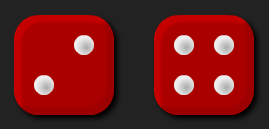#### You may also like### Chocolate

There are three tables in a room with blocks of chocolate on each. Where would be the best place for each child in the class to sit if they came in one at a time?### Four Triangles Puzzle

Cut four triangles from a square as shown in the picture. How many different shapes can you make by fitting the four triangles back together?### Cut it Out

Can you dissect an equilateral triangle into 6 smaller ones? What number of smaller equilateral triangles is it NOT possible to dissect a larger equilateral triangle into?

# Round the Dice Decimals 1

##### Age 7 to 11Challenge LevelThere are two dice, each of them with faces labelled from 1 to 6.
When the dice are rolled they can be combined in two different ways to make a number less than 10 with one decimal place.

For example, if I roll a 2 and a 3 I can combine them to make 2.3 or 3.2.

Now round each of these numbers to the nearest whole number: 2.3 rounds to 2 and 3.2 rounds to 3. Repeat for other rolls of the dice.

Do both of the numbers you make ever round to the same whole number?

There are some interactive dice here that you can use for this problem.